# Hierarchical data in Pandas

• Last Updated : 11 Dec, 2020

In pandas, we can arrange data within the data frame from the existing data frame. For example, we are having the same name with different features, instead of writing the name all time, we can write only once. We can create hierarchical data from the existing data frame using pandas.

Example:

See the student subject details. Here we can see name of student is always repeating.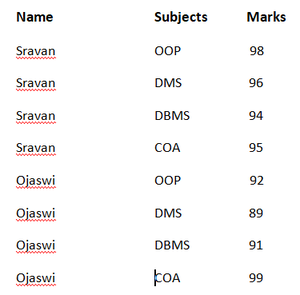With this, we need memory to store multiple name. We can reduce this by using data hierarchy.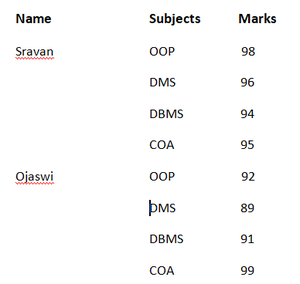Example:

## Python3

 `# import pandas  module for data frame``import` `pandas as pd`` ` `# Create dataframe for student data in different colleges``subjectsdata ``=` `{``'Name'``: [``'sravan'``, ``'sravan'``, ``'sravan'``, ``'sravan'``, ``                         ``'sravan'``, ``'sravan'``, ``'sravan'``, ``'sravan'``, ``                         ``'Ojaswi'``, ``'Ojaswi'``, ``'Ojaswi'``, ``'Ojaswi'``, ``                         ``'Ojaswi'``, ``'Ojaswi'``, ``'Ojaswi'``, ``'Ojaswi'``,``                         ``'Rohith'``, ``'Rohith'``, ``'Rohith'``, ``'Rohith'``,``                         ``'Rohith'``, ``'Rohith'``, ``'Rohith'``, ``'Rohith'``],``                 ` `                ``'college'``: [``'VFSTRU'``, ``'VFSTRU'``, ``'VFSTRU'``, ``'VFSTRU'``,``                            ``'VFSTRU'``, ``'VFSTRU'``, ``'VFSTRU'``, ``'VFSTRU'``,``                            ``'VIT'``, ``'VIT'``, ``'VIT'``, ``'VIT'``, ``'VIT'``, ``'VIT'``,``                            ``'VIT'``, ``'VIT'``, ``'IIT-Bhu'``, ``'IIT-Bhu'``, ``'IIT-Bhu'``, ``                            ``'IIT-Bhu'``, ``'IIT-Bhu'``, ``'IIT-Bhu'``, ``'IIT-Bhu'``,``                            ``'IIT-Bhu'``],``                 ` `                ``'subject'``: [``'java'``, ``'dbms'``, ``'dms'``, ``'coa'``, ``'python'``, ``'dld'``,``                            ``'android'``, ``'iot'``, ``'java'``, ``'dbms'``, ``'dms'``, ``'coa'``,``                            ``'python'``, ``'dld'``, ``'android'``, ``'iot'``, ``'java'``,``                            ``'dbms'``, ``'dms'``, ``'coa'``, ``'python'``, ``'dld'``, ``'android'``,``                            ``'iot'``]``                ``}`` ` `# Convert into data frame``df ``=` `pd.DataFrame(subjectsdata)`` ` `# print the data(student records)``print``(df)`

Output: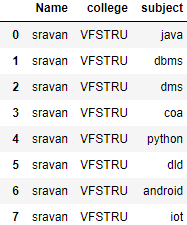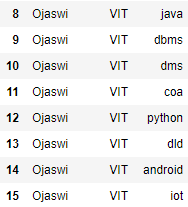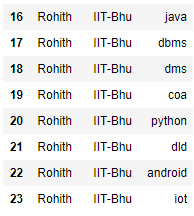## Python3

 `# Set the hierarchical index``df ``=` `df.set_index([``'Name'``, ``'college'``], drop``=``False``)`` ` `# print data frame``df`

Output: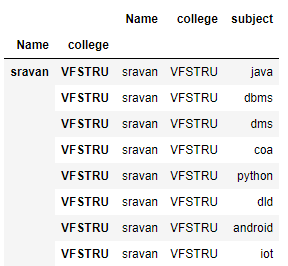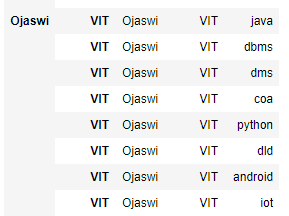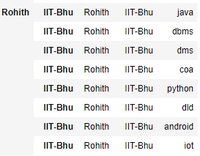The next step is to remove the name.

## Python3

 `# setting index``df ``=` `df.set_index([``'Name'``, ``'college'``])`` ` `# print data frame``df`

Output: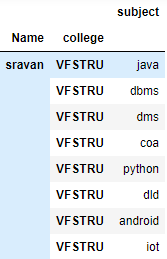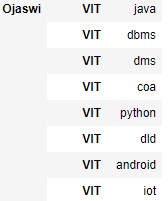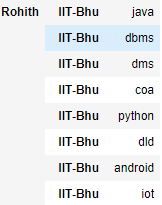Now get college as the index using swap level.

## Python3

 `# Swap the levels in the index``df.swaplevel(``'Name'``, ``'college'``)`

Output: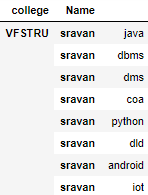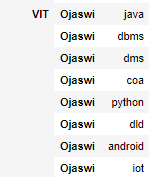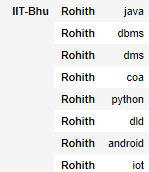Now give a summary of the results

## Python3

 `# Summarize the results by college``df.``sum``(level``=``'college'``)`

Output: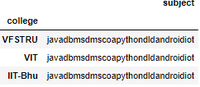My Personal Notes arrow_drop_up